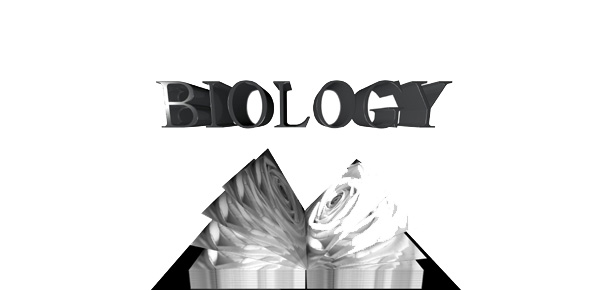# Biology And Chemistry - Mixed Science Quiz

15 Questions | Total Attempts: 598SettingsScience is distributed into different branches. Out of these, chemistry and biology are one of the most important! Where biology deals with the science of life, Chemistry is the branch that studies the behavior of matter. Take this mixed science quiz with from both biology and chemistry. ALL THE BEST!

• 1.
Choose the element that is found in all organic compounds:
• A.

Carbon

• B.

Hydrogen

• C.

Nitrogen

• D.

Oxygen

• E.

Sulphur

• 2.
The following structural formula is representative of which functional group?
• A.

Hydroxyl

• B.

Carboxyl

• C.

Sulfhydryl

• D.

Amino

• E.

Carbonyl

• 3.
Which of the functional groups illustrated below would you expect to find in an amino acid?
• A.

2 and 3

• B.

1 and 4

• C.

1, 2, and 4

• D.

3 and 5

• E.

2, 4, and 5

• 4.
In humans, the function of glycogen is to:
• A.

Dilute the blood plasma

• B.

Increase osmotic pressure in cells

• C.

Synthesize proteins

• D.

Store glucose in plants

• E.

Keep glucose available

• 5.
When a molecule of glycerol reacts with one or more fatty acids, an ester linkage results. The formation of this linkage is a result of a reaction between:
• A.

Two alcohols

• B.

Two carboxylic acids

• C.

An amino acid and a carboxylic acid

• D.

Two amino acids

• E.

An alcohol and a carboxylic acid

• 6.
When two organic molecules are joined together, and a water molecule is removed, the reaction is called which of the following?
• A.

Hydrogenation

• B.

Oxidation

• C.

Dehydration synthesis (condensation)

• D.

Reduction

• E.

Hydrolysis

• 7.
The hydrolysis (breakdown) of an ester results in:
• A.

Two acids

• B.

Two sugars

• C.

A sugar and an acid

• D.

An acid and an alcohol

• E.

An acid and an amine

• 8.
The hydrolysis (breakdown) of a dipeptide results in the production of which of the following?
• A.

Two amino acids

• B.

Two sugars

• C.

A sugar and an amino acid

• D.

An amino acid and an alcohol

• E.

An acid and an amine

• 9.
When fatty acids and glycerol chemically combine to for a fat, one other product is produced. This product is:
• A.

ATP

• B.

Water

• C.

Carbon dioxide

• D.

An amino group

• E.

A monosaccharide

• 10.
Many people are aware that water is the most abundant substance in all organisms. However, next to water, what is the major component of living cells?
• A.

Carbohydrates

• B.

Vitamins

• C.

Nucleotides

• D.

Lipids

• E.

Proteins

• 11.
Of the following, which are reasons why molecular models are useful to biologists? Models help:1. Visualize the shape of functional groups2. Visualize geometric isomers3. Visualize the three-dimensional shapes of molecules4. Determine the presence of symmetry in molecules5. Predict products of chemical reactions
• A.

1 and 2

• B.

3, 4, and 5

• C.

1 only

• D.

1, 2, 3, 4, and 5

• E.

1, 2, 4, and 5

• 12.
Which of the following is the essential characteristic of a polar molecule?
• A.

Is formed at extremely low temperatures

• B.

Has an asymmetrical distribution of electrical charge

• C.

Contains double or triple bonds

• D.

Contains the element oxygen

• E.

Contains ions as part of the structure

• 13.
Which three of the following are characteristics of hydrogen bonds?1. They are responsible for the surface tension properties of water.2. They are responsible for the relatively high boiling point of water.3. They are stronger than ionic bonds.4. They are present in all substances.5. They are weaker than covalent bonds.
• A.

1, 2, and 5

• B.

2, 3, and 4

• C.

3, 4, and 5

• D.

1, 3, and 4

• E.

1, 3, and 5

• 14.
A chemical bond involving carbon and hydrogen (C-H) is present in many biological molecules. Based upon their electronegativities, the bond could be described as:
• A.

Both ionic and covalent

• B.

A covalent bond

• C.

A weak ionic bond

• D.

A strong ionic bond

• E.

A hydrogen bond

• 15.
In terms of maintaining the shape of an enzyme, the strongest bonds involved are:
• A.

Dipole-dipole interactions

• B.

Hydrogen bonds

• C.

Covalent (disulfide) bonds

• D.

Hydrophobic bonds

• E.

Ionic interactionsBack to top